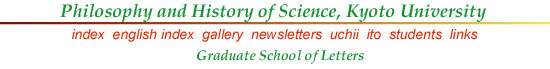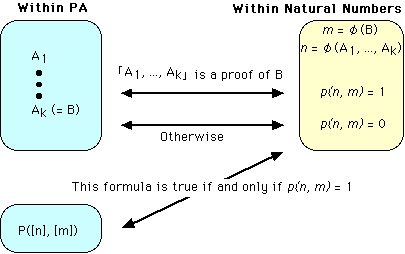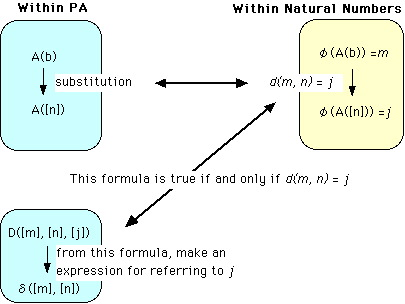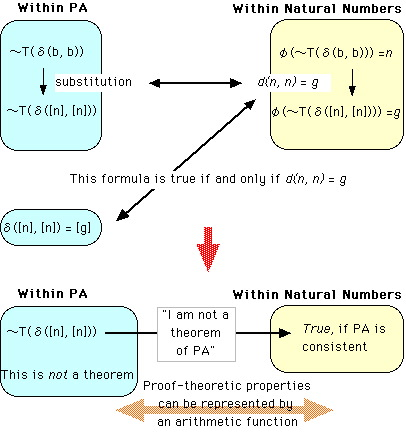Goedel's Theorem (1931)

Incompleteness Theorem in 3 pages

You already know Turing's Halting Problem, and know that it is one of the unsolvable problems for the Turing Machine. Historically, Goedel's Theorem precedes Turing's accomplishment; but it may well be the case that we can better understand Goedel's result in view of Turing's result.

Suppose an axiomatized arithmetic (number theory), called briefly Peano Arithmetic PA (see the textbook, p. 186). PA is strong enough for expressing all Turing-computable functions. Any symbol in this system, including a formula and a proof of a formula, can be represented (uniquely) by a natural number, according to Goedel's technique of Goedel numbering φ.

φ(A) = n is the Goedel number of formula (or a symbol, or a sequence) A

In the following, it is vital to distinguish clearly between (1) an arithmetic statement and (2) a formal expression for that within PA. Keeping this distinction in mind, let us define an arithmetic function p(n, m) such that:

p(n, m) = 1 if and only if n is the Goedel number of a proof of the formula which has the Goedel number m, and p(n, m) = 0 otherwise.

Corresponding to this arithmetic function, we have a formula within PA such that it can be interpreted as saying that "n is the Goedel number of a proof of the formula which has the Goedel number m". [n] is the numeral (symbol with a definite form) for number n.

P([n], [m])

That is to say, under a suitable interpretation of the symbols of PA, this formula becomes true if and only if the specified condition holds.Using this formula, we can express the concept of a "theorem of PA" as follows. A theorem of PA is a formula such that a proof exists for it. Thus a formula saying that a formula with Goedel number m is a theorem is:

∃xP(x, [m]), which may be abbreviated as T([m])

The crucial step of Godel's Theorem is a construction of another formula which can be interpreted as saying that "I am not a theorem of PA". This construction can be completed in several steps, but the point is very simple. If we can construct a formula ～T(a) such that a is a symbol which can be interpreted as referring to the Goedel number of the formula itself, then that is the formula we seek. Well, how to do that? Here, Goedel utilized the technique of diagonalization, due to Cantor, and we have already seen another version in the Halting Problem.

First, we construct an arithmetic function which computes the change of the Goedel number of a formula, when a substitution for a free variable is made. Let A(b) an arbitrary formula of PA with a free variable b. If you replace all occurrence of b by [n], you obtain formula A([n]). Now, this change by substitution is a purely mechanical change computable by a Turing machine (recall Church-Turing Thesis). Thus this change can be expressed by an arithmetic function (computable function). Let φ(A(b)) = m and φ(A([n])) = j; then, since we have a one-one correspondence between the numeral [n] and number n, the arithmetic function in question can be expressed by

d(m, n) = j

and d is computable. And since d is a function of natural numbers, it can be expressed by a formula within PA. That is, we have a formula which can be interpreted as saying that "φ(A(b)) = m and φ(A([n])) = j":

D([m], [n], [j]).From this formula, we can construct a function δ (in terms of symbols of PA) which picks out the number j satisfying this formula, such that δ(b, c) where b and c are individual variables for which an expression for designating a number can be substituted.

Now, the arithmetic function d(m, n) has two arguments; but what is going to happen if we replace n by m? d(m, m) expresses the Goedel number φ(A([m])) where φ(A(b)) = m. Here the number m plays a double role: m signifies the Goedel number on the one hand, and m corresponds to the numeral [m] on the other hand. It is here that the diagonalization comes in. By using this trick, we can construct a formula of PA which says, in effect, that "I am not a theorem of PA".

Consider the following formula:

(*)∃x(D(b, b, x)&～T(x))

and suppose the Goedel number of this formula is k. Since this formula contains a free variable b, it can be replaced by numeral [k]; then, by function d, the Goedel number of the resulting formula

(**) ∃x(D([k], [k], x)&～T(x))

is d(k, k) = i. But what does this formula say? Since the predicate D in effect expresses the function d, if d(k, k) = i then

D([k], [k], [i])

is a theorem of PA. Because of this, (**) becomes equivalent with

(***) ～T([i])

which says " the formula with Goedel number i is not a theorem of PA". But i is the Goedel number of (**) itself! Thus (**) says, in effect, "I am not a theorem of PA".

The same point can be more clearly made in terms of δ(b, b). The content of (*) can be expressed also by

(#) ～T(δ(b, b))

Supposing that the Goedel number of (#) is n, we can replace b with [n], and obtain the following formula:

(##) ～T(δ([n], [n]))

By the function d, the Goedel number of (##) is d(n, n) = g. But since δ([n], [n]) expresses d(n, n), it designate g, the Goedel number of (##). Thus (##) says "I am not a thorem of PA".Now the final step. Suppose (##) is true, i.e. arithmetically true (under the interpretation so far assumed). Then, since it says that it is not a theorem, it is not a theorem of PA. On the other hand, if it is not true, then since it says that it is not a theorem, it must be a theorem; but this is impossible if PA is consistent (if PA is consistent, no non-truth can be derived from the axioms of PA which are all true under the interpretation). Thus, the upshot is, if PA is consistent then (##) is arithmetically true but not a theorem of PA. This is what Goedel proved in 1931.